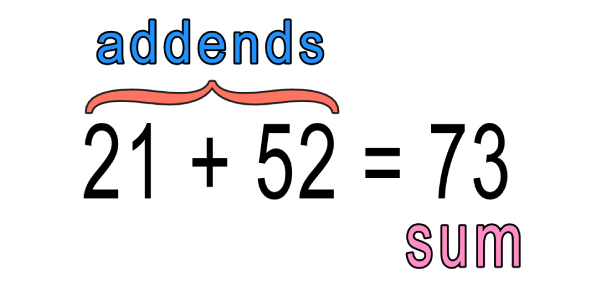10 Questions | Total Attempts: 82SettingsDo you know basic addition? Addition is one of the four basic functions of arithmetic. The other three include subtraction, multiplication, and division. The addition of whole numbers results in the total amount or sum of these values combined. Addition is putting two or more digits together to come up with a new total. Students can learn basic addition once they know how to count. Take this quiz on basic addition and see if your score adds up.

• 1.
1+1=
• A.

0

• B.

1

• C.

2

• D.

3

• 2.
2+2=
• A.

2

• B.

4

• C.

6

• D.

8

• 3.
3+3=
• A.

3

• B.

6

• C.

9

• D.

33

• 4.
4+4=
• A.

8

• B.

4

• C.

44

• D.

0

• 5.
5+5=
• A.

55

• B.

0

• C.

5

• D.

10

• 6.
6+6=
• A.

12

• B.

18

• C.

66

• D.

6

• 7.
7+7=
• A.

1

• B.

7

• C.

14

• D.

77

• 8.
8+8=
• A.

88

• B.

1

• C.

8

• D.

16

• 9.
9+9=
• A.

18

• B.

9

• C.

99

• D.

100

• 10.
10+10=
• A.

100

• B.

20

• C.

200

• D.

0

Related TopicsBack to top